# Reverse a Stack using Queue

FREE BOOK -> Problems for the day before your Coding Interview (on Amazon)In this article, we have explored the algorithm to reverse a Stack using Queue in linear time O(N). We have explained the steps along with implementation and basics of Stack and Queue.

Table of content:

1. Basics of Stack Data Structure
2. Basics of Queue Data Structure
3. Algorithm to reverse Stack using Queue
4. Pseudocode & Explanation
5. Implementation
6. Time and Space Complexity
7. Question

Let us start first of all have some idea about Stack and Queue data structure.

# Basics of Stack Data Structure

Stack is a linear data structure.It performs the operation in LIFO(Last In First Out) order. Elements are inserted at the top and are deleted from the top.Some of the real life examples of a stack are consider the bundle of a book stacked over one another in the library. In this case, we will first of all remove the book which is at the top and the book at the bottommost position will be removed at the end.In case of recursion the calling function is being stored in the stack that's why the function which is being called at the end will be returned back in the first place. It can be implemented using array and linked list both but here in this article we are going to use STL stack.Let's explore now the functions of the stack.

• push(data) : Adds an element at the top of the stack. Time Complexity : O(1)

• pop() : Removes the element from the top of the stack. Time Complexity : O(1)

• top() : Returns the reference to the top most element of the stack. Time Complexity : O(1)

• size() : Returns the size of the stack. Time Complexity : O(1)

• empty() : Returns true when the stack will be empty. Time Complexity : O(1)

push(4)
push(5)
push(6)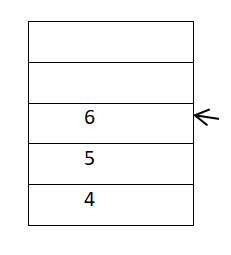pop()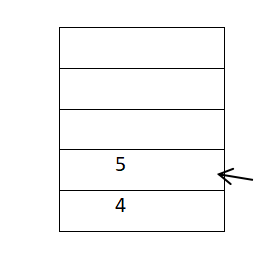Syntax of Stack in C++:

``````#include<stack>   //Header file for using STL stack.

stack<datatype> st;  //Declaration of stack.
st.push(data);       //push() function.
st.pop();            //pop() function.
bool flag = st.empty();   //empty() function.Its return type is boolean,tell us whether a stack is empty or not.
datatype tmp = st.top().  //top() function.
int s=st.size().          //size() function.
``````

# Basics of Queue Data Structure

Queue is a linear data structure. It performs the operation in FIFO (First In First Out) order. In this data structure, elements are inserted at the back and are deleted from the front. Real life example of queue is consider the queue of passengers at ticket counter, the person who reached first gets the ticket first. So,we can say that queue is opposite of stack. In queue, we remove the element the least recently added and In stack,we remove the element the most recently added.In this article we will be using STL queue.Functions of the queue are:

• push(data) : Adds the element at the end of the queue. Time Complexity : O(1)

• pop() : Removes the element from the front of the queue. Time Complexity : O(1)

• front() : Returns the reference to the front element of the queue. Time Complexity : O(1)

• size() : Returns the size of the queue. Time Complexity : O(1)

• empty() : Returns true when the queue will be empty. Time Complexity : O(1)

q.push(7)
q.push(8)
q.push(9)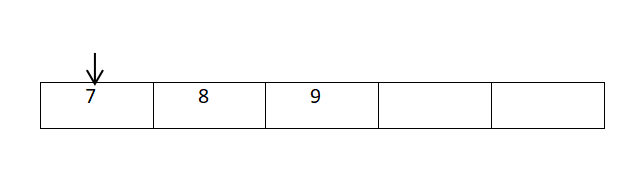q.pop()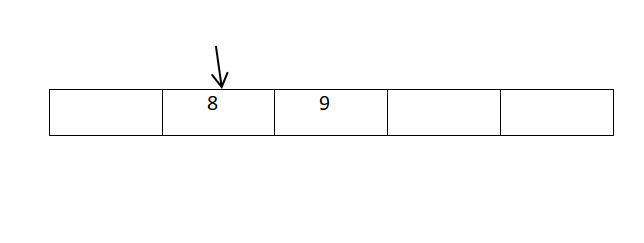Syntax of Queue in C++:

``````#include<queue>   //Header file for using STL queue.

queue<datatype> q;  //Declaration of queue.
q.push(data);       //push() function.
q.pop();            //pop() function.
bool flag = q.empty();   //empty() function.Its return type is boolean,tell us whether a queue is empty or not.
datatype tmp = q.front().  //front() function.
int s=q.size().          //size() function.
``````

# Algorithm to reverse Stack using Queue

Steps to reverse a Stack using a Queue are as follows:

1. Push all the elements into the stack.
2. Now pop the elements one by one from the stack and push it into the queue.
3. Again,push the elements into the stack.
4. Now the element in the stack will be in reverse order.

# Pseudocode & Explanation

1.First of all take input in the stack.

``````for(int i=0;i<n;i++){
cin>>data;
s.push(data);   // s is an integer stack.
}
``````

Condition of stack: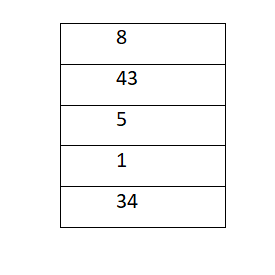1. Now,push all the elements from the stack into the queue.
``````while(s.size()!=0){
int ele=s.top();
q.push(ele);     // q is an integer queue.
s.pop();
}
``````

Condition of queue: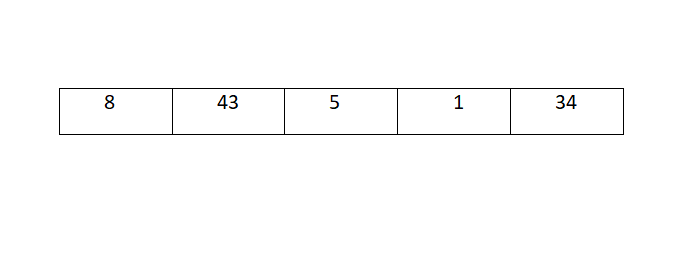Condition of stack: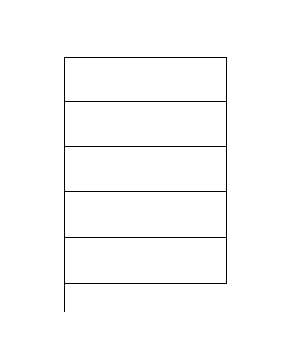1. Again,push all the elements from the queue into the stack.
``````while(q.size()!=0){
int ele=q.front();
s.push(ele);
q.pop();
}
``````

Condition of stack: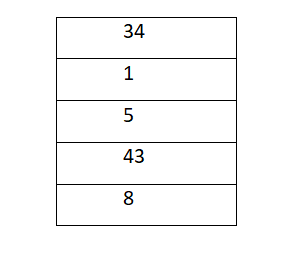# Implementation

Following is the C++ Implementation to reverse a Stack using Queue:

``````#include<iostream>
#include<stack>
#include<queue>
using namespace std;

int main(){
int n;   //Number of elements to take as input from the user.
cin>>n;

stack<int> s;
queue<int> q;
int data;
//Enter the elements.
for(int i=0;i<n;i++){
cin>>data;
s.push(data);
}

//Push the element into the queue from the stack and print the element to denote how they are being stored in the stack.

cout<<"Elements before reversing the stack:"<<endl;
while(s.size()!=0){
int ele=s.top();
cout<<ele<<" ";
q.push(ele);
s.pop();
}
cout<<endl;

//Push the element into the stack from the queue.

while(q.size()!=0){
int ele=q.front();
s.push(ele);
q.pop();
}

cout<<"Elements after reversing the stack:"<<endl;
while(s.size()!=0){
cout<<s.top()<<" ";
s.pop();
}
cout<<endl;
}
``````

Output:

``````5
34 1 5 43 8
Elements before reversing the stack:
8 43 5 1 34
Elements after reversing the stack:
34 1 5 43 8
``````

# Time and Space Complexity

• Time Complexity : O(N)
Where n is the total number of elements.

• Space Complexity: O(N)
As we have to create a Queue of N elements to reverse the original stack.

# Question

Suppose we push the elements in the stack in the order : 5,89,23,0,1.Then after reversing the stack, in which order they are being poped out from the stack.

• 5,89,23,0,1
• 1,0,23,89,5
• 0,1,5,23,89
• 1,0,5,23,89

With this article at OpenGenus, you must have the complete idea of how to reverse a Stack using Queue in linear time. Enjoy.